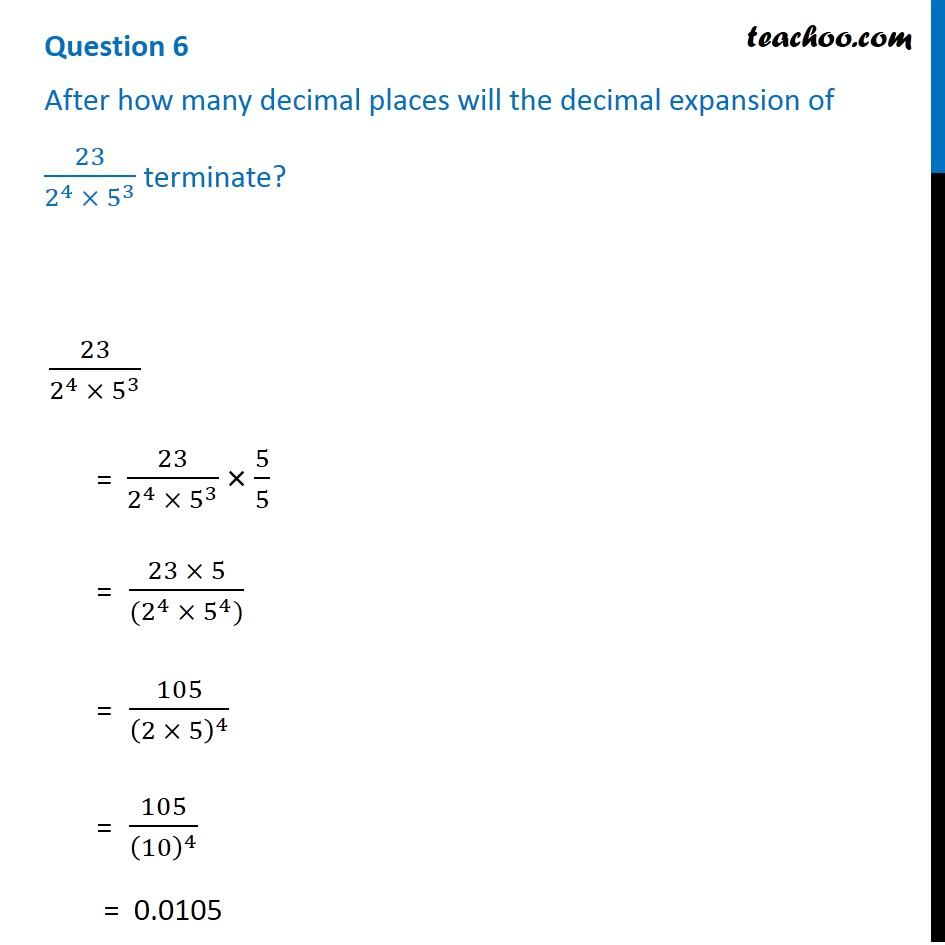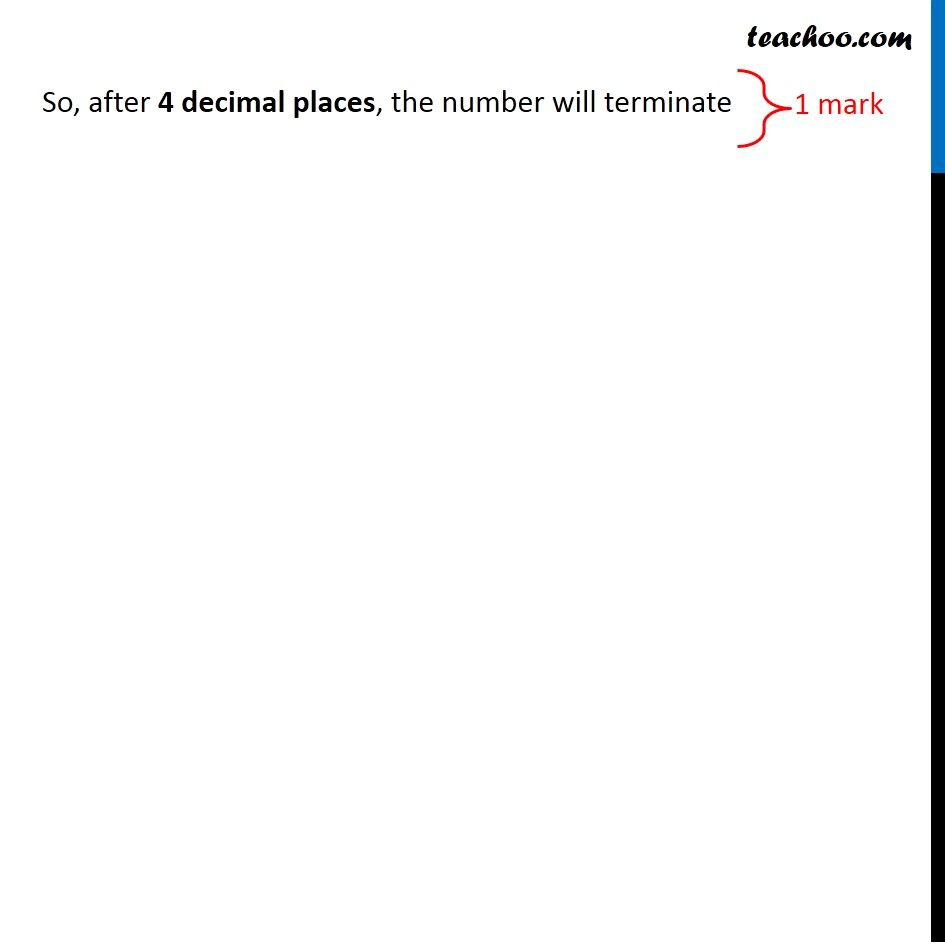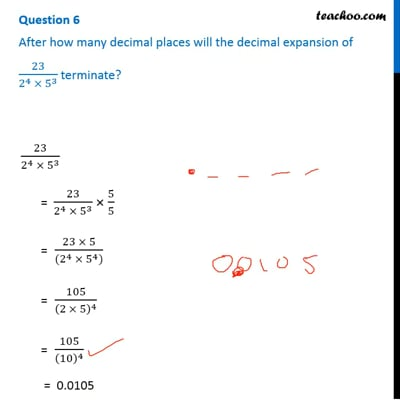CBSE Class 10 Sample Paper for 2019 Boards

Class 10
Solutions of Sample Papers for Class 10 Boards

Question 6

After how many decimal places will the decimal expansion of 23/(2 4 × 5 3 ) terminate?This video is only available for Teachoo black users

Introducing your new favourite teacher - Teachoo Black, at only ₹83 per month

### Transcript

Question 6 After how many decimal places will the decimal expansion of 23/(2^4 × 5^3 ) terminate? 23/(2^4 × 5^3 ) = 23/(2^4 × 5^3 ) × 5/5 = (23 × 5)/((2^4 × 5^4)) = 105/(2 × 5)^4 = 105/(10)^4 = 0.0105 So, after 4 decimal places, the number will terminate